# Selina Concise Solutions for Chapter 4 Cubes and Cube Roots Class 8 ICSE Mathematics

##### Exercise 4A

1. Find the cube of :
(i) 7
(ii) 11
(iii) 16
(iv) 23
(v) 31
(vi) 42
(vii) 54
Solution
(i) (7)3 = 7 × 7 × 7 = 343
(ii) (11)3 = 11 × 11 × 11 = 1331
(iii) (16)3 = 16 × 16 × 16 = 4096
(iv) (23)3 = 23 × 23 × 23 = 12167
(v) (31)3 = 31 × 31 × 31 = 29791
(iv) (42)3 = 42 × 42 × 42 = 74088
(vii) (54)3 = 54 × 54 × 54 = 157464

2. Find which of the following are perfect cubes:
(i) 243
(ii) 588
(iii) 1331
(iv) 24000
(v) 1728
(vi) 1938
Solution
(i) 243∵ 243 = 3 × 3 × 3 × 3
= (3 × 3 × 3) × 3
= 33 × 3
∴ 297 is not a perfect cube.

(ii) 588588 = 2 × 2 × 7 × 7 × 3
∴ 588 is not a perfect cube.

(iii)
1331∵ 1331 = 11 × 11 × 11 = (11)3
∴ 1331 is perfect cube.

(iv) 24000
∵  24000 = 2 × 2 × 2 × 2 × 2 × 2 × 3 × 5 × 5 × 5
= (2)3 × (2)3 × (5)3 × 3
∴  24000 is not a perfect cube.

(v) 1728∵ 1728 = 2 × 2 × 2 × 2 × 2 × 2 × 2 × 3 × 3 × 3
= (2)3 × (2)3 × (3)3
∴ 1728 is a perfect cube.

(vi) 1938
1938 = 2 × 3 × 17 × 19
1938 is not a perfect cube.

3. Find the cubes of :
(i) 2.1
(ii) 0.4
(iii) 1.6
(iv) 2.5
(v) 0.12
(vi) 0.02
(vii) 0.8
Solution
(i) 2.1
= (2.1)3
= (21/10)3
= (21 ×  21 ×  21)/(10 × 10 × 10)
= 9261/1000
= 9.261

(ii) 0.4
= (0.4)3
= (4/10)3
= (4 × 4 × 4)/(10 × 10 × 10)
= 64/1000
= 0.064

(iii) 1.6
= (1.6)3
= (16/10)3
= (16 × 16 × 16)/(10 × 10 × 10)
= 4096/1000
= 4.096

(iv) 2.5
= (2.5)3
= (25/10)3
= (25 × 25 × 25)/(10 × 10 × 10)
= 15625/1000
= 15.625

(v) 0.12
= (0.12)3
= (12/100)3
= (12 × 12 × 12)/(100 × 100 × 100)
= 1728/1000000
= 0.001728

(vi) 0.02
= (0.02)3
= (2/100)3
= (2 × 2 × 2)/(100 × 100 × 100)
= 8/1000000
= 0.000008

(vii) 0.8
= (0.8)3
= (8/10)3
= (8 × 8 × 8)/(10 × 10 × 10)
= 512/100
= 0.512

4. Find the cubes of:
(i) 3/7
(ii) 8/9
(iii) 10/13
(iv) 1 2/7
(v) 2 ½
Solution
(i) 3/7
= (3/7)3
= (3 × 3 × 3)/(7 × 7 × 7)
= 27/343

(ii) 8/9
= (8/9)3
= (8 × 8 × 8)/(9 × 9 × 9)
= 512/729

(iii) 10/13
= (10/13)3
= (10 × 10 × 10)/(13 × 13 × 13)
= 1000/2197

(iv) 1 2/7
= (1 2/7)3
= {(1 × 7 + 2)/7}3
= (9/7)3
= (9 × 9 × 9)/(7 × 7 × 7)
= 729/343
= 2 43/343

(v)
= (2 1/2)3
= (5/2)3
= (5 × 5 × 5)/(2 × 2 × 2)
= 125/8
= 15 5/8

5. find the cubes of :
(i) -3
(ii) -7
(iii) -12
(iv) -18
(v) -25
(vi) -30
(vii) -50
Solution
(i) -3
= (-3)3
= -3 × -3 × -3
= -(3 × 3 × 3)
= -27

(ii) -7
= (-7)
= -7 × -7 × -7
= -(7 × 7 × 7)
= -343

(iii) -12
= (-12)3
= -12 × -12 × -12
= -(12 × 12 ×12)
= -1728

(iv) -18
= (-18)3
= -18 × -18 × -18
= -(18 × 18 × 18)
= -5832

(v) -25
= (-25)3
= -25 × -25 × -25
= -(25 × 25 × 25)
= -15625

(vi) -30
= (-30)3
= -30 × -30 × -30
= -(30 × 30 × 30)
= -27000

(vii) -50
= (-50)3
= -50 × -50 × -50
= -(50 × 50 × 50)
= -125000

6. Which of the following are cubes of:
(i) an even number
(ii) an odd number
216, 729, 3375, 8000, 125, 343, 4096 and 9261.
Solution
∵ 216 = 2 × 2 × 2 × 3 × 3 × 3= (2)3 × (3)3 = (6)3

∵  729 = 3 × 3 × 3 × 3  × 3 ×  3= (3)3 × (3)3 = (9)3

∵  3375 = 5 × 5 × 5 × 3 × 3 × 3= (5)3 × (3)3 = (15)3

∵  8000 = 20 × 20 × 20 = (20)3125 = 5 × 5 × 5 = (5)3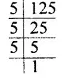∵  343 = 7 × 7 × 7 = (7)3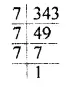∵  4096 = 2 × 2 × 2 × 2 ×  2 ×  2 × 2 × 2 × 2 × 2 × 2 × 2= (2)3 × (2)3 × (2)3 × (2)3 = (16)3
(i) Cubes of an even number are 216, 8000, 4096.
(ii) Cubes of an odd number are 729, 3375, 125, 343, 9261.

7. Find the least number by which 1323 must be multiplied so that the product is a perfect cube.
Solution
The prime factor of 1323 are = 3 × 3 × 3 × 7 × 7
= (3 × 3 × 3) × 7 × 7
Clearly, 1323 must be multiplied by 7.

8. Find the smallest number by which 8768 must be divided so that the quotient is a perfect cube.
Solution
The prime factor 8768 are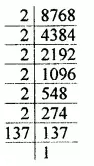= 2 × 2 × 2 × 2 × 2  × 2 × 137
= (2 × 2 × 2) × (2 × 2 × 2) × 137
Clearly, 8768 must be divided by 137.

9. Find the smallest number by which 27783 be multiplied to get a perfect square number.
Solution
= 3 × 3 × 3 × 3 × 7 × 7 × 7
= (3 × 3 × 3) × (7 × 7 × 7) × 3
Clearly, 27783 must be multiplied by 3 × 3 = 9

10. With what least number must 8640 be divided so that the quotient is a perfect cube?
Solution
The prime factor of 8640 are= 2 ×  2 × 2 × 2 × 2 × 2 × 3 × 3 × 3 ×  5
= (2 × 2 × 2) × (2 × 2 × 2) × (3 × 3 × 3) × 5
Clearly, 8640 must be divided by 5.

11. Which is the smallest number that must be multiplied to 77175 to make it a perfect cube?
Solution
The prime factors of 77175 are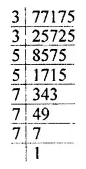= 3 × 3 × 5 × 5 × 7 × 7 × 7
= (7 × 7 × 7) × 3 × 3 × 5 × 5
Clearly, 77175 must be multiplied by 3 × 5 = 15

### Exercise 4 B

1. Find the cube-roots of :
(i) 64
(ii) 343
(iii) 729
(iv) 1728
(v) 9261
(vii) 4096
(vii) 8000
(viii) 3375
Solution
(i)(ii)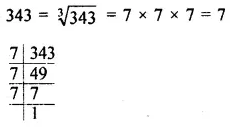(iii) 729 = ∛729  = (3 × 3 × 3) × (3 × 3 × 3)
= 3 × 3
= 9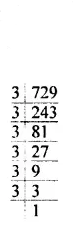(iv)(v)(vi)(vii)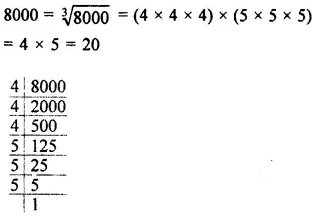(viii)2. Find the cube-roots of :
(i) 27/64
(ii) 125/216
(iii) 343/512
(iv) 64 × 729
(v) 64 × 27
(vi) 729 × 8000
(vii) 3375 × 512
Solution
(i)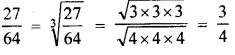(ii)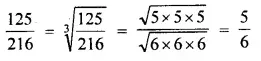(iii)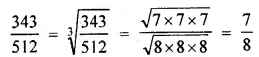(iv)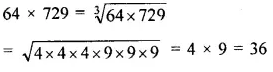(v)(vi)(vii)3. Find the cube-roots of :
(i) -216
(ii) -512
(iii) -1331
(iv)  -27/125
(v) -64/343
(vi)  -512/343
(vii) -2197
(viii) -5832
(ix) -2744000
Solution
(i)(ii)(iii)(iv)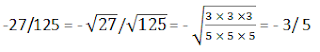(v)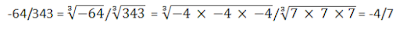(vi)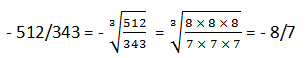(vii)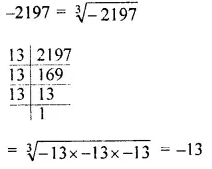(viii)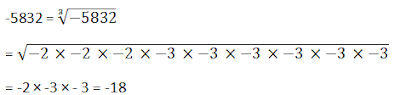(ix)4. Find the cube-roots of :
(i) 2.744
(ii) 9.261
(iii) 0.000027
(iv) -0.512
(v) -15.625
(vi) -125 x 1000
Solution
(i)(ii)(iii)(iv)(v)(vi)5. Find the smallest number by which 26244 may be divided so that the quotient is a perfect cube.
Solution
The prime factors of 26244 are= 2 × 2 × 3 × 3  × 3 × 3 × 3 × 3 × 3 × 3
= (3 × 3 × 3) × (3 × 3 × 3) × 3 × 3 × 2 × 2
Clearly, 26244 must be divided by 3 × 3 × 2 × 2 = 36

6. What is the least number by which 30375 should be multiplied to get a perfect cube?
Solution
The prime factors of 30375 are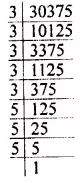= 3 × 3 × 3 × 3 × 3 × 5 × 5 × 5
= (3 × 3 × 3) × (5 × 5 × 5) × 3 × 3
clearly, 30375 must be  multiplied with 3

7. Find the cube-roots of :
(i) 700 x 2 x 49 x 5
(ii) -216 x 1728
(iii) -64 x -125
(iv) -27/343
(v) 729/-1331
(vi) 250.047
(vii) -175616
Solution
(i) 700 × 2 × 49 × 5= 2 × 2 5 × 5 × 7 × 2 × 7 × 7 × 5
= (2 × 2 × 2) × (5 × 5 × 5) × (7 × 7 × 7)
= 2 × 5 × 10 = 70

(ii) -216 × 1728= -(2 × 2 × 2 × 3 × 3 × 3) × (2  × 2 × 2 × 2 × 2 × 2 × 3 × 3 × 3)
= -2 × 3 × 2 × 2 × 2 × 3
= -72

(iii) -64 × -125
= -(4 × 4 × 4) × -(5 × 5 × 5)
= -4 × -5
= 20

(iv) - 27/343
= (3 × 3 × 3)/(7 × 7 × 7)
= -3/7

(v) 729/-1331
= (9 × 9 × 9)/-(11 × 11 ×11)
= - 9/11

(vi) 250.047 = 250047/1000= {(3 × 3 × 3) × (3 × 3 × 3) × (7 × 7 × 7)/(10 × 10 × 10)}
= (3 × 3 × 7)/10
= 63/10
= 6.3

(vii) -175616= -[(2 × 2 × 2) × (2 × 2 × 2) × (2 × 2 × 2) × (7 × 7 × 7)]
= -[2 × 2 × 2 × 7]
= -56